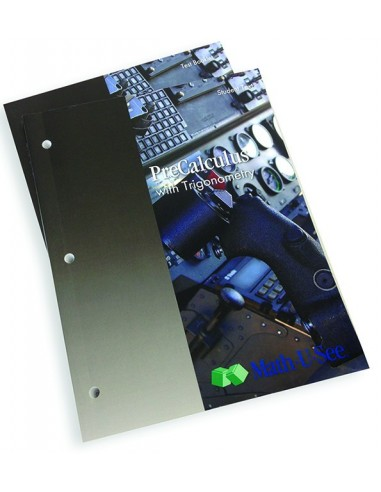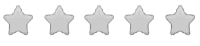See inside this book

# Math-U-See PreCalculus Student Pack

\$43.00
No tax
Demme Learning
Math
12
P-MA12-62Leave a Review

#### Description

New! Math-U-See PreCalculus Student Pack

Math-U-See is a complete, skill-based, multi-sensory homeschool math curriculum for grades K-12 that?s designed to teach students skills in a logical sequence.

The PreCalculus Student Pack contains the Student Workbook with lesson-by-lesson worksheets and honors pages and the PreCalculus Tests.

Math-U-See PreCalculus covers Trigonometry, Identities, Polar Equations, Logarithms, Sequences, Limits and other topics to prepare for Calculus.

Pythagorean Theorem Special Right Triangles (30?- 60?- 90? and 45?- 45?- 90?) Trigonometric Ratios Inverse Trigonometric Ratios Interpreting the Trigonometry Tables Using the Trig. Table to Solve for the Unknown Using a Calculator and Arc Functions Angles of Elevation and Depression Angles less than 0? Angles greater than 360? Reference Angles Cofunctions Negative Angle Relationships Proving Trigonometric Identities Sum and Difference Identities Double-Angle and Half-Angle Identities Law of Sines Law of Cosines Ambiguity in the Law of Sines Radian Measure Polar Coordinates and Rectangular Coordinates Polar Equations and Polar Graphs Vectors Functions: Relation, Domain, and Range Graphing the Sine and Cosine Functions Graphing the Secant and Cosecant Functions Graphing the Tangent and Cotangent Functions Logarithms Arithmetic Sequences and Series Geometric Sequences and Series Proof of Cos (A - B) = Cos A Cos B + Sin A Sin B Finding the Area of a Triangle Trigonometrically Interpolation Navigation Natural Logarithms Equations with Absolute Value Equations with Radicals Inequalities with Absolute Value Inequalities with Radicals Limits